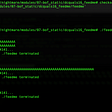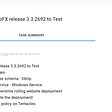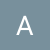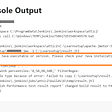# Implement HashMap in Java

`private class HMNode{      K key;      V value;     HMNode(K key, V value){       this.key=key;       this.value=value;     }   }`
`public static class HashMap<K,V>{   private class HMNode{      K key;      V value;     HMNode(K key, V value){       this.key=key;       this.value=value;     }   }  private int size=n;  private LinkedList<HMNode>[] buckets; //--> length of bucket= N  public HashMap(){    size=0;    initbuckets(8);  }  private initbuckets(int N){    buckets= new LinkedList[N];    for(int i=0;i<N;i++){      buckets[i] =new LinkedList();    }  }}`
`private int hashfn(K key){    int hc= key.hashcode();    return Math.abs(hc)%buckets.length;  }  public int getIndexWithinBucket(K key, int bi){    int di=0;    for(HMNode node: buckets[bi]){      if(node.key.equals(key){        return di;      }         di++;    }     return -1;  }  public void put(K key, V value){    int bi = hashfn(key);    int idx = getIndexWithinBucket(key,bi);    if(di!=-1){      HMNode node = buckets[bi].get(idx);      node.value= value;    }    else{      HMNode node = new HMNode(key,value);      buckets[bi].add(node);      size++;    }    double lambada = size*1.0/buckets.length;    if (lambda>2.0) {        rehash();      //here 2.0  is threshold;    }      }  public void rehash(){  LinkedList<HMNode> temp[] = buckets;  buckets= initbuckets(2*buckets.length);  int size=0;  for(int i=0;i<temp.length;i++){    for(HMNode node: temp[i]){      put(node.key,node.value);    }  } }`
`public V getKey(K key) throws Exception{    int bi = hashfn(key);    int idx = getIndexWithinBucket(key,bi);   if(idx!=-1){    HMNode node = buckets[bi].get(idx);    return node.value   }   else return null; }`
`public boolean containsKey(K key) throws Exception{    int bi = hashfn(key);    int idx = getIndexWithinBucket(key,bi);   if(idx==-1) return false;   else return true; }`
`public V remove(K key) throws Exception{      int bi = hashfn(key);    int idx = getIndexWithinBucket(key,bi);   if(idx!=-1){    HMNode node = buckets[bi].remove(idx);     size--;    return node.value   }   else return null; }`
`public int size(){  return size; }`
`public ArrayList<K> keySet(){    ArrayList<K> keys= new ArrayList();   for(int i=0;i<buckets.length;i++){    for(HMNode node: buckets[i]){      keys.add(node.key);    }   }   return keys; }`

--

--

--

## More from Abhinay Gupta

Love podcasts or audiobooks? Learn on the go with our new app.

## Machine Learning on Containers!## 3 interview examples to check that developer can understand business## 5 Steps to easy web Scraping## DEFCON 2016 Quals writeup — feedme## Octopus: Automation Software Deployment Tool## Using multiple player prefabs with UNET in Unity## 5 Reasons To Learn Python Right Now## Govrn Community Update — August 2021## Solution for “Not able to find Java executable or version” in Jenkins## Introduction to Parallel Programming in Java## Why Learn Java in 2021## Java History